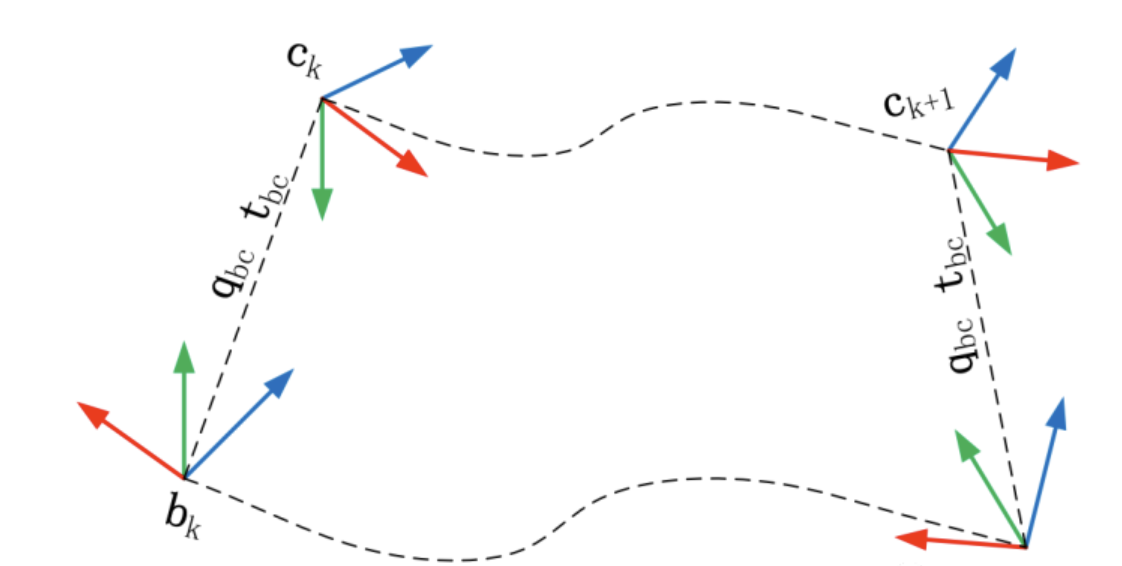# VINS 中的旋转外参初始化

​ 为了使这个两个传感器融合，我们首先需要做的事情是将两个传感器的数据对齐，除了时间上的对齐，还有空间上的对齐。空间上的对齐通俗的讲就是将一个传感器获取的数据统一到另一个传感器的坐标系中，其关键在于确定这两个传感器之前的外参，本文将详细介绍 VINS_Monocamera-imu 的旋转外参标定算法原理并对其代码进行解读（VINS-Fusion中也是一样的）。

​ 相机与 IMU  之间的相对旋转如下图所示：​ 上图表示相机和 IMU 集成的系统从到的运动，其中视觉可以通过特征匹配求得到时刻的旋转增量，同时 IMU 也可以通过积分得到到时刻的旋转增量。两个求得的旋转增量应当是相同的，因此分别将在自己坐标系下求得的增量转移到对方的坐标系下，即经过 $$\mathbf{q}_{bc}$$ 变换后应当还是相同的。

​ 相邻两时刻 $$k$$ , $$k + 1$$ 之间有: IMU 旋转积分 $$\mathbf{q}_{b_{k} b_{k+1}}$$ ,视觉测量 $$\mathbf{q}_{c_{k} c_{k+1}}$$。则有:

$\mathbf{q}_{b_{k} b_{k+1}} \otimes \mathbf{q}_{b c}=\mathbf{q}_{b c} \otimes \mathbf{q}_{c_{k} c_{k+1}}$

​ 上面的式子可以写成：

$\left(\left[\mathbf{q}_{b_{k} b_{k+1}}\right]_{L}-\left[\mathbf{q}_{c_{k} c_{k+1}}\right]_{R}\right) \mathbf{q}_{b c}=\mathbf{Q}_{k+1}^{k} \cdot \mathbf{q}_{b c}=\mathbf{0}$

​ 其中 $$\left[ \dot{\ \ \ } \right]_L, \left[ \dot{\ \ \ } \right]_R$$ 表示  left and right quaternion multiplication，不清楚可以看附录，其实就是四元数乘法换了个表达形式。

​ 将多个时刻线性方程累计起来，并加上鲁棒核权重得到:

$\begin{array}{c} {\left[\begin{array}{c} w_{1}^{0} \cdot \mathbf{Q}_{1}^{0} \\ w_{2}^{1} \cdot \mathbf{Q}_{2}^{1} \\ \vdots \\ w_{N}^{N-1} \cdot \mathbf{Q}_{N}^{N-1} \end{array}\right] \mathbf{q}_{b c}=\mathbf{Q}_{N} \cdot \mathbf{q}_{b c}=\mathbf{0}} \\ w_{k+1}^{k}=\left\{\begin{array}{ll} 1, & r_{k+1}^{k}<\text { threshold } \\ \frac{\text { threshold }}{r_{k+1}^{k}}, & \text { otherwise } \end{array}\right. \end{array}$

• 代码
bool InitialEXRotation::CalibrationExRotation(vector<pair<Vector3d, Vector3d>> corres, Quaterniond delta_q_imu, Matrix3d &calib_ric_result)
{
frame_count++;
Rc.push_back(solveRelativeR(corres));                               // 对极几何计算出的 R,t 约束
Rimu.push_back(delta_q_imu.toRotationMatrix());     // IMU 预积分的得到的 R,t 约束
Rc_g.push_back(ric.inverse() * delta_q_imu * ric);       // 将imu预积分转换到相机坐标系

Eigen::MatrixXd A(frame_count * 4, 4);
A.setZero();
int sum_ok = 0;
for (int i = 1; i <= frame_count; i++)
{
Quaterniond r1(Rc[i]);
Quaterniond r2(Rc_g[i]);

// A.angularDistance(B):是求两个旋转之间的角度差，用弧度表示
double angular_distance = 180 / M_PI * r1.angularDistance(r2);
ROS_DEBUG(
"%d %f", i, angular_distance);

double huber = angular_distance > 5.0 ? 5.0 / angular_distance : 1.0; // huber 核函数作加权
++sum_ok;
// L R 分别为左乘和右乘矩阵
Matrix4d L, R;

double w = Quaterniond(Rc[i]).w();
Vector3d q = Quaterniond(Rc[i]).vec();
L.block<3, 3>(0, 0) = w * Matrix3d::Identity() + Utility::skewSymmetric(q);
L.block<3, 1>(0, 3) = q;
L.block<1, 3>(3, 0) = -q.transpose();
L(3, 3) = w;

Quaterniond R_ij(Rimu[i]);
w = R_ij.w();
q = R_ij.vec();
R.block<3, 3>(0, 0) = w * Matrix3d::Identity() - Utility::skewSymmetric(q);
R.block<3, 1>(0, 3) = q;
R.block<1, 3>(3, 0) = -q.transpose();
R(3, 3) = w;

A.block<4, 4>((i - 1) * 4, 0) = huber * (L - R);
}

// svd 分解中最小奇异值对应的右奇异向量作为旋转四元数
JacobiSVD<MatrixXd> svd(A, ComputeFullU | ComputeFullV);
Matrix<double, 4, 1> x = svd.matrixV().col(3);
Quaterniond estimated_R(x);
// 所求的 x 是q^b_c，在最后需要转换成旋转矩阵并求逆。
ric = estimated_R.toRotationMatrix().inverse();
//cout << svd.singularValues().transpose() << endl;
//cout << ric << endl;
Vector3d ric_cov;
ric_cov = svd.singularValues().tail<3>();
// 至少迭代计算了WINDOW_SIZE次，且R的奇异值大于0.25才认为标定成功
if (frame_count >= WINDOW_SIZE && ric_cov(1) > 0.25)
{
calib_ric_result = ric;
return true;
}
else
return false;
}



## Appendix

• The product of two quaternions is bi-linear and can be expressed as two equivalent matrix products, namely
$\mathbf{q}_{1} \otimes \mathbf{q}_{2}=\left[\mathbf{q}_{1}\right]_{L} \mathbf{q}_{2} \quad \text { and } \quad \mathbf{q}_{1} \otimes \mathbf{q}_{2}=\left[\mathbf{q}_{2}\right]_{R} \mathbf{q}_{1},$

$$\quad$$where $$[\mathbf{q}]_{L}$$ and $$[\mathbf{q}]_{R}$$ are respectively the left- and right- quaternion-product matrices

$[\mathbf{q}]_{L}=\left[\begin{array}{cccc} q_{w} & -q_{x} & -q_{y} & -q_{z} \\ q_{x} & q_{w} & -q_{z} & q_{y} \\ q_{y} & q_{z} & q_{w} & -q_{x} \\ q_{z} & -q_{y} & q_{x} & q_{w} \end{array}\right], \quad[\mathbf{q}]_{R}=\left[\begin{array}{cccc} q_{w} & -q_{x} & -q_{y} & -q_{z} \\ q_{x} & q_{w} & q_{z} & -q_{y} \\ q_{y} & -q_{z} & q_{w} & q_{x} \\ q_{z} & q_{y} & -q_{x} & q_{w} \end{array}\right],$

$$\quad$$then

$[\mathbf{q}]_{L}=q_{w} \mathbf{I}+\left[\begin{array}{cc} 0 & -\mathbf{q}_{v}^{\top} \\ \mathbf{q}_{v} & {\left[\mathbf{q}_{v}\right]_{\times}} \end{array}\right], \quad[\mathbf{q}]_{R}=q_{w} \mathbf{I}+\left[\begin{array}{cc} 0 & -\mathbf{q}_{v}^{\top} \\ \mathbf{q}_{v} & -\left[\mathbf{q}_{v}\right]_{\times} \end{array}\right]$

## VINS中旋转外参初始化的更多相关文章

1. VINS（四）初始化与相机IMU外参标定

和单目纯视觉的初始化只需要获取R,t和feature的深度不同,VIO的初始化话通常需要标定出所有的关键参数,包括速度,重力方向,feature深度,以及相机IMU外参$R_{c}^{b}$和$p_{ ... 2. VI ORB-SLAM初始化与VINS初始化对比(将vi orb-slam初始化方法移植到vins中) 初始化时需要求出的变量:相机和imu外参r t.重力g.尺度s.陀螺仪和加速度计偏置ba bg. 下面对两种算法初始化的详细步骤进行对比: 求陀螺仪偏置bg 求解公式相同,求解方法不同.公式如下,VI ... 3. VINS 估计器之外参初始化 为何初始化外参 当外参完全不知道的时候,VINS也可以在线对其进行估计(rotation),先在processImage内进行初步估计,然后在后续优化时,会在optimize函数中再次优化. 如何初始 ... 4. 相机-imu外参校准总结 1. 研究背景及相关工作 1)研究背景 单目视觉惯性slam是一种旨在跟踪移动平台的增量运动并使用来自单个车载摄像头和imu传感器的测量结果同时构建周围环境地图的技术.视觉相机和惯性测量单元(imu) ... 5. 相机imu外参标定 1. 第一步初始化imu外参(可以从参数文档中读取,也可以计算出),VINS中处理如下: # Extrinsic parameter between IMU and Camera. estimate_ ... 6. 解放双手——相机与IMU外参的在线标定 本文作者 沈玥伶,公众号:计算机视觉life,编辑部成员 一.相机与IMU的融合 在SLAM的众多传感器解决方案中,相机与IMU的融合被认为具有很大的潜力实现低成本且高精度的定位与建图.这是因为这两个 ... 7. C++类中成员变量的初始化总结（转帖） 本文转自:C++类中成员变量的初始化总结 1. 普通的变量: 一般不考虑啥效率的情况下 可以在构造函数中进行赋值.考虑一下效率的可以再构造函数的初始化列表中进行. 1 class CA ... 8. 关于map容器的元素被无参初始化 使用C++中的map容器定义一个mp,当你执行if语句判断mp是否为1时,那么如果mp以前不存在,此时mp就会被无参初始化,second赋值为0. 以下的程序可以证明这一点.执行了第 ... 9. C++类中成员变量的初始化总结 @import url(http://i.cnblogs.com/Load.ashx?type=style&file=SyntaxHighlighter.css);@import url(/c ... 10. 虚机中访问外网；NAT中的POSTROUTING是怎么搞的？ 看下docker中是怎么配置的网络 在虚机中访问外网:设定了qemu,在主机上添加路由:sudo iptables -t nat -I POSTROUTING -s 192.168.1.110 -j ... ## 随机推荐 1. Django-账号用户密码修改 Django账号密码修改命令: python manage.py changepassword python manage.py changepassword 实操分析: 第一次修改失败是因为违反了密 ... 2. Docker-Compose快速搭建LNMP Docker-Compose 1.安装Docker sudo apt -y install docker.io docker version 查看版本号 docker help 查看帮助文档 2.更换 ... 3. Docker 的安装及常用命令 CentOS Docker 安装 参看链接 Windows安装 Docker Desktop 官方下载地址: https://hub.docker.com/editions/community/doc ... 4. Vue3从入门到精通(二) vue3 侦听器 在Vue3中,侦听器的使用方式与Vue2相同,可以使用watch选项或$watch方法来创建侦听器.不同之处在于,Vue3中取消了immediate选项,同时提供了新的选项和API. ...

5. API NEWS | Money Lover爆出潜在API漏洞

欢迎大家围观小阑精心整理的API安全最新资讯,在这里你能看到最专业.最前沿的API安全技术和产业资讯,我们提供关于全球API安全资讯与信息安全深度观察. 本周,我们带来的分享如下: Money Lov ...

6. 深入分析：Lasso问题和原子范数问题研究

写在前面 本文将主要围绕Lasso问题和原子范数等经典问题进行对偶问题的推导.分析,由于笔者的数理基础浅薄,下面的证明过程若存在错误,欢迎评论指正. LASSO问题 推导 ​ 问题定义:\(\unde ...

7. 解决NAT模式下SSH连接虚拟机

解决NAT模式下SSH连接虚拟机 简介: 用到的有软件:VirtualBox6.1,RetHat7.4 , SmartTTY 来由: 刚开始使用桥接模式(Bridged)网络连接,但是虚拟机没有网络. ...

8. 【论文阅读】Pyramid Scene Parsing Network

解决的问题:(FCN) Mismatched Relationship: 匹配关系错误,如将在水中的船识别为车. Confusion Categories: 模糊的分类,如 hill 和 mounta ...

9. AI隐私保护中的常见隐私隐私问题与解决方案

目录 题目:<AI隐私保护中的常见隐私问题与解决方案> 引言 随着人工智能技术的快速发展,AI隐私保护也成为了一个备受关注的问题.由于AI技术的应用范围越来越广泛,例如语音识别.图像识别. ...

10. PHP curl提交参数到某个网址，然后获取数据

<?php $data = '你的每个参数';$url = 'https://www.bz80.vip/'; //举例 $html = post_data($url,\$data); echo ...